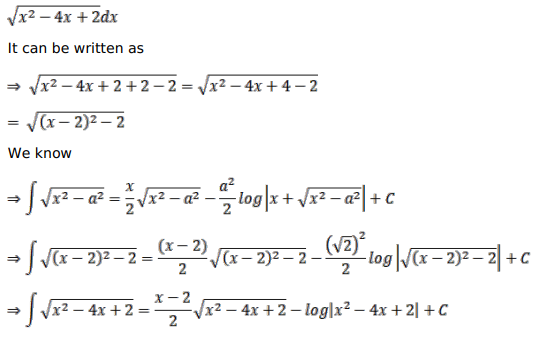# Solve this followingQuestion:

Mark $(\sqrt{)}$ against the correct answer in each of the following:

$\int \sqrt{x^{2}-4 x+2} d x=?$

A. $\frac{1}{2}(x-2) \sqrt{x^{2}-4 x+2}+\log \left|(x-2)+\sqrt{x^{2}-4 x+2}\right|+C$

B. $(x-2) \sqrt{x^{2}-4 x+2}+\frac{1}{2} \log \left|(x-2)+\sqrt{x^{2}-4 x+2}\right|+C$

C. $\frac{1}{2}(x-2) \sqrt{x^{2}-4 x+2}-\log \left|(x-2)+\sqrt{x^{2}-4 x+2}\right|+C$

D. none of these

Solution: